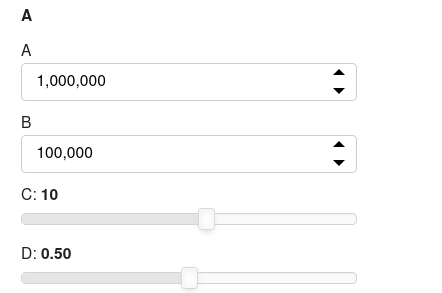# Overridding default value format in param panel

Is there a better way to override the default value formatting for numeric panel input widgets?

``````import numbers
from bokeh.models.formatters import NumeralTickFormatter
import param as pm
import panel as pn
pn.extension()

class A(pm.Parameterized):
a = pm.Number(1000000, step=10000)
b = pm.Number(100000, step=1000)
c = pm.Number(10, step=1, bounds=(-100,100))
d = pm.Magnitude(0.5)

def widgets(self):
number_params = [k for k,v in self.param.values().items() if isinstance(v, numbers.Number) and v > 100]
number_format = {"format": NumeralTickFormatter(format="‘0,0’")}
widgets={a: number_format for a in number_params}
return widgets

a = A()

pn.Param(a, widgets=a.widgets())
``````Cheers

1 Like

Didn’t occur to me that you could use bokeh formatting on those widgets, but ofc you can!The NumeralTickFormatter you’re referring to has several other formats:

If you use FuncTickFormatter, you can use your own JS code and add e.g. engineering notation.

``````JS_ENG_UNITS = """
var space = ' ';
var space2 = '    ';

// B
if (Math.abs(tick) >= 1e8 && Math.abs(tick) < 1e11){
return space2 + (tick / 1e9).toFixed(2) + space + 'B';
}
// M
if (Math.abs(tick) >= 1e5 && Math.abs(tick) < 1e8){
return space2 + (tick / 1e6).toFixed(2) + space + 'M';
}
// k
if (Math.abs(tick) >= 1e2 && Math.abs(tick) < 1e5){
return space2 + (tick / 1e3).toFixed(2) + space + 'k';
}
// 1 - 100
if (Math.abs(tick) >= 1e0 && Math.abs(tick) < 1e2){
return space2 + (tick / 1e0).toFixed(2) + space;
}
// 1e-3 - 1
//if (Math.abs(tick) >= 1e-3 && Math.abs(tick) < 1e0){
//    return space2 + (tick).toFixed(3) + space;
//}
// 1e-3 - 1e-2
if (Math.abs(tick) >= 1e-3 && Math.abs(tick) < 1e0){
return space2 + (tick * 1e3).toFixed(2) + space + 'm';
}
// 1e-6 - 1e-3
if (Math.abs(tick) >= 1e-6 && Math.abs(tick) < 1e-2){
return space2 + (tick * 1e6).toFixed(2) + space + 'µ';
}
// 1e-9 - 1e-6
if (Math.abs(tick) >= 1e-9 && Math.abs(tick) < 1e-5){
return space2 + (tick * 1e9).toFixed(2) + space + 'n';
}
// zero
if (Math.abs(tick) == 0){
return space2 + tick.toFixed(2) + space;
}
// outside bounds
else{
return space2 + tick.toExponential() + space;
}
"""

formatter = FuncTickFormatter(code=JS_ENG_UNITS)
``````

For anyone interested I created a Parameterized baseclass that offers the formatting method that I proposed and additionally auto generates the step value for numeric parameters to be about 1% of their bounds range if they have bounds, or 1% of their default value if they don’t have bounds.

Cheers1 Like